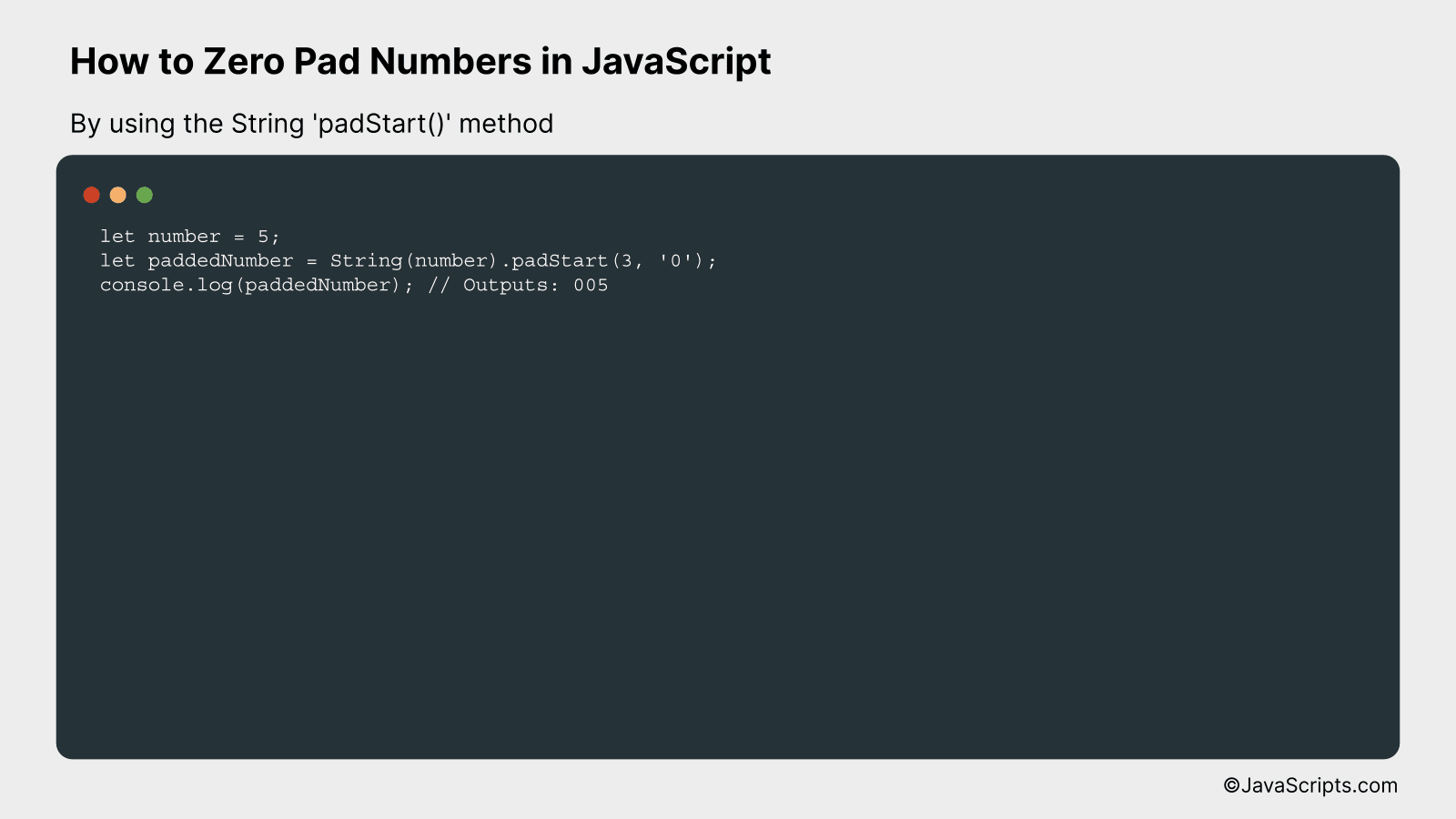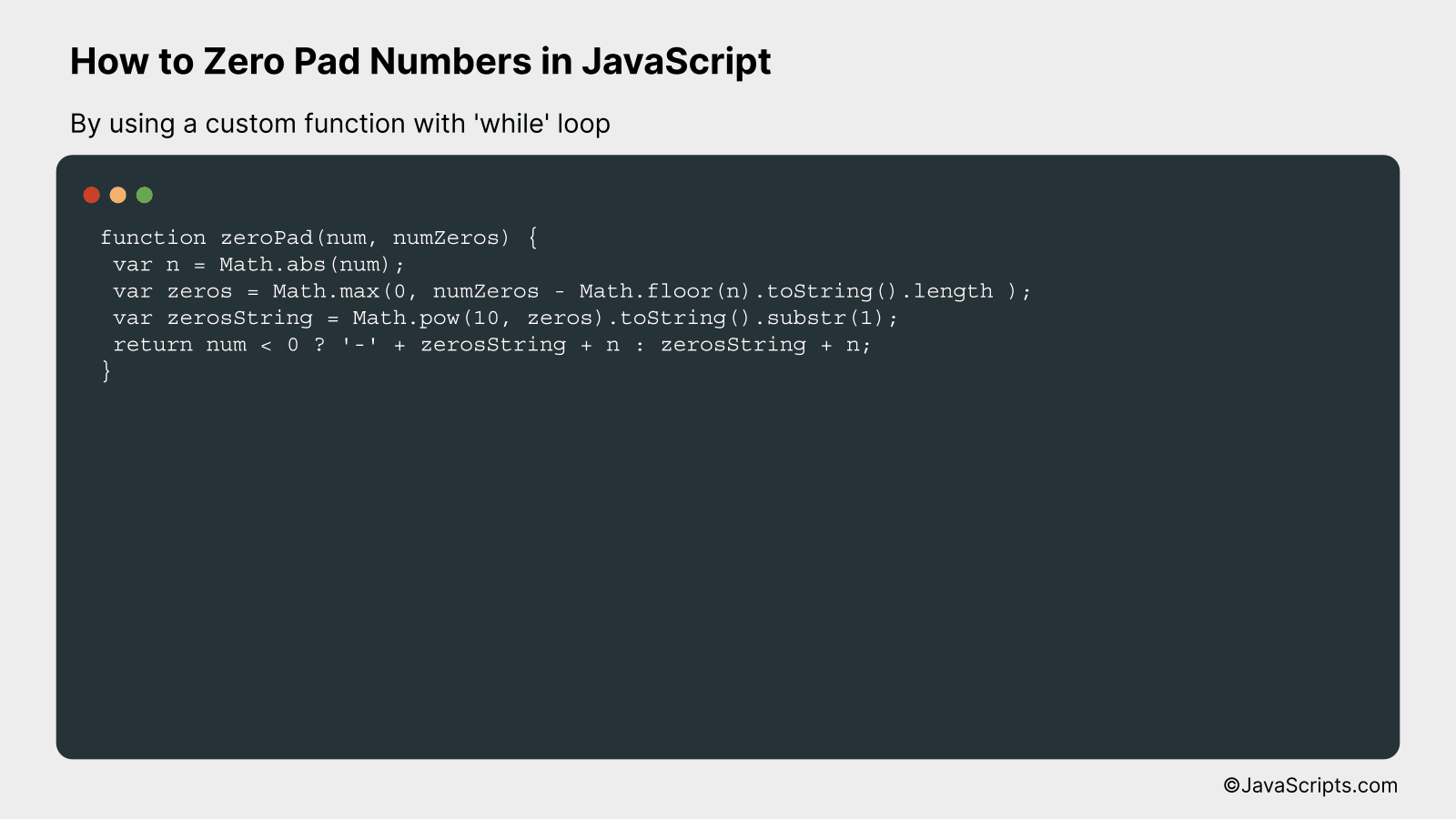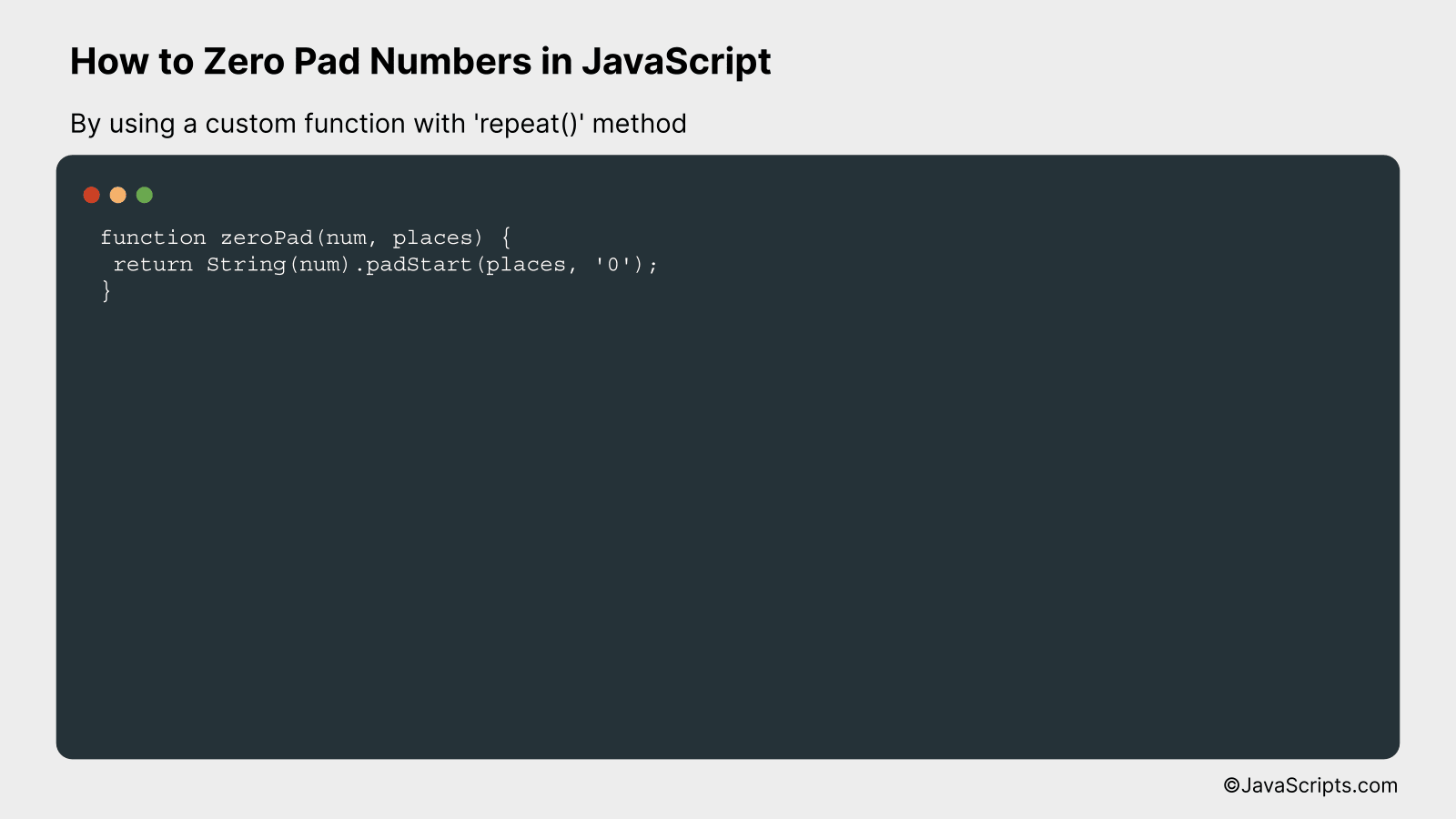# How to Zero Pad Numbers in JavaScript

There’s a common coding challenge often encountered by JavaScript developers: zero padding numbers. If you’re new to this term, it might sound technical, but it’s actually a straightforward concept. Zero padding means adding zeros before a number to make it a certain length.

Say you’re working on a digital clock application, and you want to display single digit numbers as two digits (i.e., ’08’ instead of ‘8’). Or maybe you’re handling product codes in an inventory system that require six digits, with leading zeros if necessary. That’s where zero padding becomes incredibly useful. Together, let’s dive into how to tackle this with JavaScript.

In this post, we will be looking at the following 3 ways to zero pad numbers in JavaScript:

• By using the String ‘padStart()’ method
• By using a custom function with ‘while’ loop
• By using a custom function with ‘repeat()’ method

Let’s explore each…

## #1 – By using the String ‘padStart()’ methodThe ‘padStart()’ method in JavaScript is a built-in method that pads the current string from the start with a specified string (the second argument) until the resulting string reaches the given length (the first argument). If you have a number and you want to pad it to be of a specific length, you can convert the number into a string and then use the ‘padStart()’ method. For example, if you have the number ‘5’ and you want it to always be three digits (i.e., ‘005’), you can use ‘padStart()’ to achieve this.

``````
let number = 5;
``````

### How it works

The ‘padStart()’ method is used to pad a string from the start (left) to reach a certain length. It takes two arguments: the final length of the string and the string to use for padding. In this case, we’re padding the string representation of a number with ‘0’s so it always has at least three digits.

• First, we define a number, let’s say ‘5’ in this case.
• We convert this number into a string using the ‘String()’ method because ‘padStart()’ is a string method.
• We then use the ‘padStart()’ method on this string, specifying ‘3’ as the final desired length and ‘0’ as the padding string.
• If our string is less than three characters long, ‘padStart()’ will add ‘0’s to the start of the string until it is three characters long.
• Finally, we log ‘paddedNumber’ to the console, which will output ‘005’.

## #2 – By using a custom function with ‘while’ loopThis custom JavaScript function will prepend zeros to a given number until it reaches a specified length. For instance, if we pad the number ‘9’ to a length of ‘5’, the output will be ‘00009’.

``````
var n = Math.abs(num);
var zeros = Math.max(0, numZeros - Math.floor(n).toString().length );
var zerosString = Math.pow(10, zeros).toString().substr(1);
return num < 0 ? '-' + zerosString + n : zerosString + n;
}
```
```

### How it works

The function zeroPad takes two parameters: the number to be padded num and the total length of the desired string numZeros. The function calculates the needed number of zeros and prepends them to the given number, returning the padded number as a string.

Here's a step-by-step breakdown:

• The function takes in the number and the target length as input.
• It calculates the absolute value of the input number to ensure padding works for negative numbers as well.
• It then computes the current length of the number and subtracts it from the target length to find out how many zeros are needed.
• It creates a string of zeros of the required length.
• Finally, the function checks if the initial number was negative. If it was, it returns the zero-padded string with a '-' sign at the start. Otherwise, it just returns the zero-padded string.

## #3 - By using a custom function with 'repeat()' methodThis JavaScript function works by converting the input number into a string and using the 'repeat()' method to prepend zeros until the desired length is reached. For example, if you input (5,3), the output will be '005'.

``````
}
```
```

### How it works

This function takes a number and the total number of digits you want the output to have. It then converts the number to a string and uses the 'padStart()' method to add zeros in front of the number until the string reaches the specified length.

• 'num' is the number you want to pad with zeros.
• 'places' is the total length you want the output string to be.
• First, the number is converted to a string using the 'String()' function.
• Then, the 'padStart()' method is used on this string. This method pads the current string with another string (second argument) until the resulting string reaches the given length (first argument).
• The padding is applied from the start (left) of the current string.